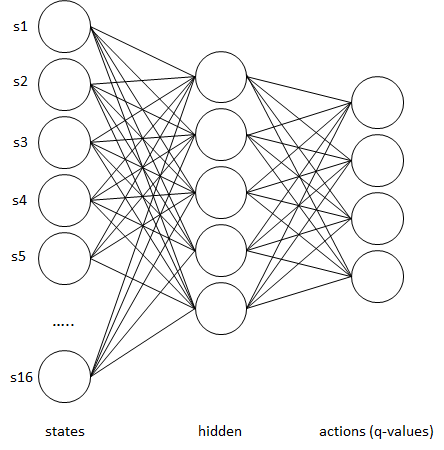# Refactoring FrozenLake to Deep Reinforcement Learning

I am really into reinforcement learning at the moment. For me it is a phantastic approach for training an AI. In the beginning, I had some trouble understanding deep q-learning compared to plain q-learning. Many tutorials go fast and start implementing deep q-learning using a CNN to solve games like doom. This is very funny, but makes it neccessary to understand CNN’s as well deeply. To get a more intuitive understanding, I wanted to implement a simpler problem that is also solvable with q-learning. View this great tutorial about q-learning first: https://medium.freecodecamp.org/diving-deeper-into-reinforcement-learning-with-q-learning-c18d0db58efe

So the idea of going deep is that the q-table gets too big, because a lot of state information is involved. Imagine the state space of a big problem. Let’s say we want to find the optimal path for delivering packets. We could assume a simplified area of 128×128 fields. We could have 20 packets to collect and to deliver. This would already result in a state space of 128x128x20x20 = 6.553.600. The actions we would imply, is simply left, up, right, down. This would result in a q-table with 6.553.600 x 4 = 26.214.400 entries.

For larger problems, this table is not storable into memory anymore. So the idea for deep q-learning is to combine deep neural networks with reinforcement learning. The q-table gets approximated by a neural network! So the idea of this tutorial is to refactor the FrozenLake problem from a q-learning approach to a deep-q-learning approach. It would be super if you did the q-learning tutorial before at https://medium.freecodecamp.org/diving-deeper-into-reinforcement-learning-with-q-learning-c18d0db58efe.

So for the frozen lake problem, we had a state space of 16, because there is a 4×4 grid involved. The actions we can do are left, up, right, down. The initalized q-table results in a 4×16 one, where the 16 rows are the states, and the 4 columns are the actions. The values are the reward, we get for a certain action:

```    L U R D
S1 [0 0 0 0]
S2 [0 0 0 0]
.........```

Instead of generating this table, we could use a neural network instead:The inputs are our states, the outputs are the actual table entries. The neural network can learn these entries. To get the best action, we take the maximal q-value from the output layer. The following example is the frozen lake problem solved using deep learning, and the original file using a q-table.